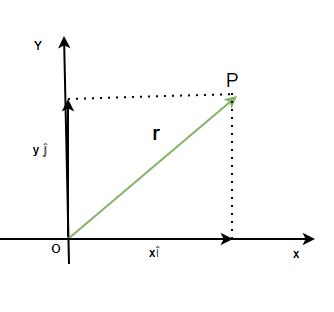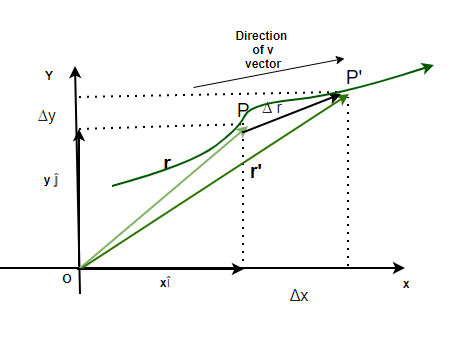Average Velocity

• Last Updated : 30 Jun, 2021

It is essential to know how to describe the motion of a body or a particle. These conventions and methods allow us to formalize and then use them to develop further theory. Speed is the notion of how fast a particle is moving. It’s used almost every day and denotes the rate of change of position of an object. In theory, it is essential to describe the speed and the direction of the particle in a formal way. This is done using vectors and this is called velocity. Let’s look at this concept in detail.

Motion in a Plane

When a particle moves from one point to another point on the X and Y-axis. It is said to be moving on a plane. A plane is made up of X and Y axes, which are used to denote the position of the particle. Motion in a plane is also described in terms of the X and Y coordinates of the particles and how fast they are changing. The motion can be plotted on a Cartesian plane as shown in the figure below.The motion of any particle moving in a plane can be described using its position and its velocity.

Position Vector

The position vector is used to denote the position of the particle on the Cartesian plane with respect to the origin as a reference. The position vectorfor a particle is given by,Where x and y are their components along x and y-axis.

Suppose a particle moves fromto. Then the displacement is directed towards,Velocity

The average velocity is the ratio of total displacement over total time. Suppose a particle goes fromtoin a total time ofThe velocity is given by,This assumes that the velocity of an object is constant, but this is often not the case. Sometimes, velocity changes with time. In that case, instantaneous velocity should be calculated. It is given by,The direction of velocity of a particle following a path at any point is given by a tangent drawn at that particular point in the direction of motion. Velocity can also be expressed in the form of its components.So, if the components of a velocity are known, the magnitude of velocity can be calculated using,And the direction is given by angle,Let’s look at some sample problems.

Sample Problems

Question 1: Find the displacement vector for the particle which is moving in a plane and whose position vectors are given below,

vi = 3i + 4j and vf = 5i + 2j

Given: the initial and final position vectors,

vi = 3i + 4j

v = 5i + 2j

The goal is find the displacement vector. It is given by,HereandPlugging these values in the equation,Question 2: Find the displacement vector for the particle which is moving in a plane and whose position vectors are given below,

vi = i + j and vf = 2i + 5j

Given: the initial and final position vectors,

vi = i + j

v = 2i + 5j

The goal is to find the displacement vector. It is given by,HereandPlugging these values into the equation,Question 3: Find the velocity at t = 4, for the particle which is moving in a plane and whose position is given below,

r = 2t2i + t3j

Given: the initial and final position vectors,

r = 2t2i + t3j

The position vector changes with time. The velocity in this case is given by the formula,Here x(t) = 2t2 and y(t) = t3

Plugging these values into the equation,At t = 4,Question 4: Find the velocity at t = 2, for the particle which is moving in a plane and whose position is given below,

r = ti + 4t2j

Given: the initial and final position vectors,

r = ti + 4t2j

The position vector changes with time. The velocity in this case is given by the formula,Here x(t) = t and y(t) = 4t2

Plugging these values into the equation,at t = 4,Question 5: Find the average velocity between t = 0 and t = 2, for the particle which is moving in a plane and whose position is given below,

r = 3ti + 3t3j

Given: the initial and final position vectors,

r = 3ti + 3t3j

The position vector changes with time. The average velocity is given by the formula,At t = 0

r = 0i + 0j

At t = 2

r’ = 6i + 24jPlugging the values in this above equation,Question 6: Find the average velocity between t = 1 and t = 4, for the particle which is moving in a plane and whose position is given below,

r = ti + tj

Given: the initial and final position vectors,

r = ti + tj

The position vector changes with time. The average velocity is given by the formula,At t = 1

r = 1i + 1j

At t = 4

r’ = 4i + 4jPlugging the values in this above equation,My Personal Notes arrow_drop_up